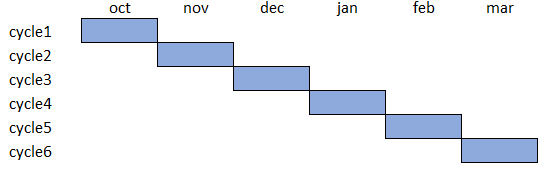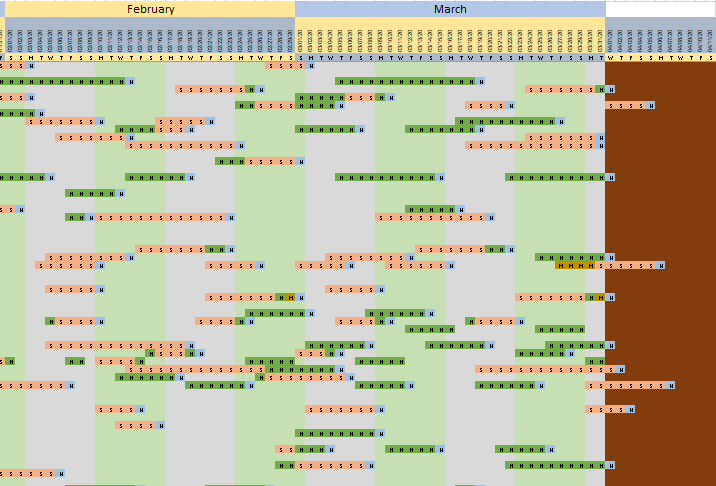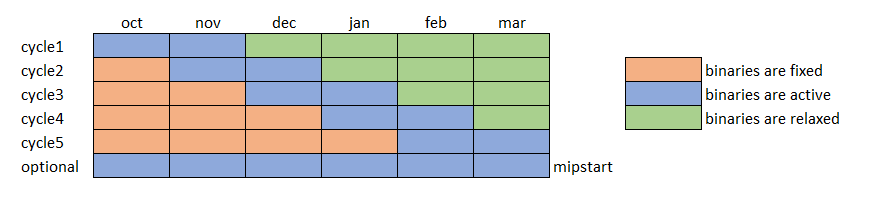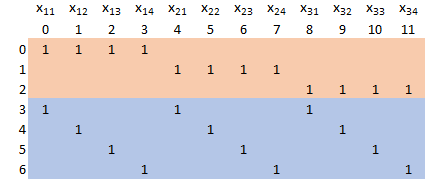## Wednesday, October 23, 2019

### Rolling horizon approach for scheduling model

I need to run a (large) scheduling model with a planning horizon of several months: october 2019 through march 2020. This leads to a very large MIP model. One way to split this up into smaller problems is to solve it one month at the time.Simple approach

This is easier said than done. We don't have a nice break in the schedule at the end of the month. Assignments are bleeding into the next month:Schedule (partial view)

One way to deal with this problem is to shift the window a bit more complicated way:Tailored rolling horizon algorithm

We basically solve the problem as a MIP two months at the time, but shift it by only one month. The binary variables more in the future are relaxed to continuous variables. That part becomes like an LP. I.e. the green parts are easy. Past binary variables are fixed. Of course, the MIP presolver will remove all fixed variables from the model, so the orange parts are also easy.

This approach only really works if we don't have global constraints over all months. The danger is that we push the bad stuff into the future. That can even lead to infeasible sub-problems at the end.  Luckily this model has no constraints that span all six months.

If we want we can solve the big model at the end using the solution we built up in parts as a starting point (using the mipstart option). If the algorithm is working as expected, this last big MIP model should not find solutions that are much better. This is indeed the case for my model. (Note that not all sub-problems are solved to optimality -- sometimes a small gap remains).

## Sunday, October 13, 2019

### Sometimes a commercial solver is really better...

Solving a model with an integer valued objective, with 500 binary variables, gave some interesting results.

• Cplex. Optimal solution $$z=60$$ found in about 8 seconds. Using 4 threads on an old laptop. Log is below.
• CBC. Hit timelimit of 2 hours. Objective = 62 (non-optimal). Also using 4 threads on the same machine. The strange thing is that with CBC the best possible bound is not changing at all. Not by a millimeter. See the highlighted numbers in the log below. CBC is a very good solver, but sometimes I see things like this.
• Gurobi and Xpress solve this problem very fast. Ran on NEOS via an MPS file.
• SCIP has also problems with this model. Ran on NEOS via an MPS file.

All runs were with default settings. It looks like the commercial solvers do a much better job than the open source and academic codes. Sometimes you get what you pay for.

#### Cplex Log

Tried aggregator 2 times.
MIP Presolve eliminated 4 rows and 5 columns.
MIP Presolve modified 3 coefficients.
Aggregator did 500 substitutions.
Reduced MIP has 999 rows, 1499 columns, and 2497 nonzeros.
Reduced MIP has 500 binaries, 0 generals, 0 SOSs, and 0 indicators.
Presolve time = 0.02 sec. (7.38 ticks)
Found incumbent of value 500.000000 after 0.03 sec. (8.85 ticks)
Probing time = 0.00 sec. (0.20 ticks)
Tried aggregator 1 time.
Reduced MIP has 999 rows, 1499 columns, and 2497 nonzeros.
Reduced MIP has 500 binaries, 0 generals, 0 SOSs, and 0 indicators.
Presolve time = 0.00 sec. (5.26 ticks)
Probing time = 0.00 sec. (0.19 ticks)
MIP emphasis: balance optimality and feasibility.
MIP search method: dynamic search.
Parallel mode: deterministic, using up to 4 threads.
Parallel mode: deterministic, using up to 3 threads for concurrent optimization.
Tried aggregator 1 time.
LP Presolve eliminated 999 rows and 1499 columns.
All rows and columns eliminated.
Presolve time = 0.02 sec. (0.71 ticks)
Initializing dual steep norms . . .
Root relaxation solution time = 0.02 sec. (1.15 ticks)

Nodes                                         Cuts/
Node  Left     Objective  IInf  Best Integer    Best Bound    ItCnt     Gap

*     0+    0                          500.0000        0.0000           100.00%
Found incumbent of value 500.000000 after 0.06 sec. (16.78 ticks)
0     0       55.2664   490      500.0000       55.2664        0   88.95%
*     0+    0                           75.0000       55.2664            26.31%
Found incumbent of value 75.000000 after 0.08 sec. (21.35 ticks)
0     0       55.2825   353       75.0000     Cuts: 349      222   26.29%
0     0       55.4160   311       75.0000     Cuts: 349      455   26.11%
0     0       55.4160   275       75.0000     Cuts: 349      775   26.11%
*     0+    0                           72.0000       55.4160            23.03%
Found incumbent of value 72.000000 after 0.59 sec. (116.10 ticks)
0     0       55.4160   182       72.0000     Cuts: 349     1032   23.03%
0     0       55.4160   141       72.0000     Cuts: 349     1208   23.03%
0     0       55.4160   121       72.0000     Cuts: 192     1336   23.03%
0     0       55.4160   105       72.0000     Cuts: 128     1410   23.03%
0     0       55.4160    98       72.0000      Cuts: 85     1472   23.03%
0     0       55.4160    61       72.0000      Cuts: 74     1494   23.03%
0     0       55.4160    67       72.0000     Cuts: 160     1571   23.03%
0     2       55.4160    56       72.0000       55.4160     1571   23.03%
Elapsed time = 1.33 sec. (273.54 ticks, tree = 0.01 MB, solutions = 3)
1239   854       55.4160    59       72.0000       55.4160     3845   23.03%
Cuts: 38
*  1471+ 1230                           71.0000       55.4160            21.95%
Cuts: 16
Found incumbent of value 71.000000 after 3.39 sec. (827.52 ticks)
*  1474+ 1230                           70.0000       55.4160            20.83%
Found incumbent of value 70.000000 after 3.39 sec. (828.63 ticks)
*  1481+ 1230                           69.0000       55.4160            19.69%
Found incumbent of value 69.000000 after 3.41 sec. (831.79 ticks)
*  1490+ 1230                           68.0000       55.4160            18.51%
Found incumbent of value 68.000000 after 3.44 sec. (834.87 ticks)
*  1731+ 1033                           67.0000       58.7491            12.31%
Found incumbent of value 67.000000 after 6.63 sec. (1824.17 ticks)
*  1731+  688                           65.0000       59.0933             9.09%
Found incumbent of value 65.000000 after 7.25 sec. (2079.31 ticks)
*  1731+  458                           62.0000       60.0000             3.23%
Found incumbent of value 62.000000 after 7.47 sec. (2162.68 ticks)
*  1731+  305                           61.0000       60.0000             1.64%
Found incumbent of value 61.000000 after 8.20 sec. (2495.45 ticks)
*  1731+    0                           60.0000       60.0000             0.00%
Found incumbent of value 60.000000 after 8.58 sec. (2603.40 ticks)
*  1731     0      integral     0       60.0000       60.0000    10141    0.00%
Found incumbent of value 60.000000 after 8.61 sec. (2604.50 ticks)

Cover cuts applied:  150
Implied bound cuts applied:  14
Flow cuts applied:  37
Mixed integer rounding cuts applied:  249
Gomory fractional cuts applied:  26

Root node processing (before b&c):
Real time             =    1.31 sec. (273.18 ticks)
Real time             =    7.30 sec. (2331.56 ticks)
Sync time (average)   =    0.25 sec.
Wait time (average)   =    0.01 sec.
------------
Total (root+branch&cut) =    8.61 sec. (2604.75 ticks)
MIP status(101): integer optimal solution


#### CBC Log

Calling CBC main solution routine...
Integer solution of 74 found by feasibility pump after 0 iterations and 0 nodes (2.78 seconds)
Integer solution of 72 found by RINS after 0 iterations and 0 nodes (2.96 seconds)
At root node, 128 cuts changed objective from 55.26645 to 55.416026 in 10 passes
Cut generator 0 (Probing) - 367 row cuts average 2.1 elements, 0 column cuts (12 active)  in 0.022 seconds - new frequency is 1
Cut generator 1 (Gomory) - 598 row cuts average 26.1 elements, 0 column cuts (0 active)  in 0.090 seconds - new frequency is 1
Cut generator 2 (Knapsack) - 14 row cuts average 11.9 elements, 0 column cuts (0 active)  in 0.037 seconds - new frequency is -100
Cut generator 3 (Clique) - 0 row cuts average 0.0 elements, 0 column cuts (0 active)  in 0.003 seconds - new frequency is -100
Cut generator 4 (MixedIntegerRounding2) - 368 row cuts average 11.2 elements, 0 column cuts (0 active)  in 0.023 seconds - new frequency is 1
Cut generator 5 (FlowCover) - 394 row cuts average 2.8 elements, 0 column cuts (0 active)  in 0.041 seconds - new frequency is 1
Cut generator 6 (TwoMirCuts) - 598 row cuts average 35.5 elements, 0 column cuts (0 active)  in 0.072 seconds - new frequency is -100
After 0 nodes, 1 on tree, 72 best solution, best possible 55.416026 (3.94 seconds)
Integer solution of 70 found by heuristic after 4138 iterations and 57 nodes (14.24 seconds)
Integer solution of 69 found by heuristic after 12712 iterations and 287 nodes (23.32 seconds)
Integer solution of 66 found by heuristic after 18149 iterations and 487 nodes (25.94 seconds)
After 1005 nodes, 555 on tree, 66 best solution, best possible 55.416026 (31.73 seconds)
Integer solution of 65 found by heuristic after 32591 iterations and 1015 nodes (33.93 seconds)
Integer solution of 64 found by heuristic after 43252 iterations and 1405 nodes (39.79 seconds)
Integer solution of 63 found by heuristic after 52409 iterations and 1805 nodes (44.62 seconds)
After 2017 nodes, 1104 on tree, 63 best solution, best possible 55.416026 (45.77 seconds)
After 3045 nodes, 1653 on tree, 63 best solution, best possible 55.416026 (52.29 seconds)
After 4099 nodes, 2212 on tree, 63 best solution, best possible 55.416026 (55.02 seconds)
After 5163 nodes, 2769 on tree, 63 best solution, best possible 55.416026 (57.08 seconds)

. . .

After 131221 nodes, 37229 on tree, 63 best solution, best possible 55.416026 (610.94 seconds)
After 132282 nodes, 37230 on tree, 63 best solution, best possible 55.416026 (613.18 seconds)
After 133298 nodes, 37231 on tree, 63 best solution, best possible 55.416026 (615.50 seconds)
After 134320 nodes, 37318 on tree, 63 best solution, best possible 55.416026 (621.40 seconds)
Integer solution of 62 found by heuristic after 2839018 iterations and 134502 nodes (621.99 seconds)
After 135334 nodes, 37422 on tree, 62 best solution, best possible 55.416026 (627.07 seconds)
After 136352 nodes, 37407 on tree, 62 best solution, best possible 55.416026 (631.83 seconds)
After 137391 nodes, 37395 on tree, 62 best solution, best possible 55.416026 (637.74 seconds)
After 138400 nodes, 37385 on tree, 62 best solution, best possible 55.416026 (642.25 seconds)

. . .

After 1566320 nodes, 37408 on tree, 62 best solution, best possible 55.416026 (7177.35 seconds)
After 1567325 nodes, 37412 on tree, 62 best solution, best possible 55.416026 (7182.33 seconds)
After 1568336 nodes, 37421 on tree, 62 best solution, best possible 55.416026 (7187.07 seconds)
After 1569358 nodes, 37402 on tree, 62 best solution, best possible 55.416026 (7192.93 seconds)
After 1570410 nodes, 37400 on tree, 62 best solution, best possible 55.416026 (7199.07 seconds)
Thread 0 used 30010 times,  waiting to start 2696,  0 locks, 0 locked, 0 waiting for locks
Thread 1 used 30010 times,  waiting to start 2480,  0 locks, 0 locked, 0 waiting for locks
Thread 2 used 30010 times,  waiting to start 2083,  0 locks, 0 locked, 0 waiting for locks
Thread 3 used 30010 times,  waiting to start 1770,  0 locks, 0 locked, 0 waiting for locks
Main thread 6737 waiting for threads,  0 locks, 0 locked, 0 waiting for locks
Exiting on maximum time
Partial search - best objective 62 (best possible 55.416026), took 19832056 iterations and 1570713 nodes (7202.49 seconds)
Strong branching done 3540964 times (6897 iterations), fathomed 137838 nodes and fixed 779663 variables
Maximum depth 198, 2057372 variables fixed on reduced cost

Time limit reached. Have feasible solution.
MIP solution:    6.200000e+01   (1570713 nodes, 7202.62 CPU seconds, 7202.62 wall clock seconds)


## Wednesday, October 9, 2019

### The gas station problem: where to pump gas and how much

#### Problem

The problem (from ) is to determine where to pump gasoline (and how much) during a trip, where prices between gas stations fluctuate.

We consider some different objectives:

• Minimize Cost. This results in an LP model.
• Minimize Number of Stops. This makes the model a MIP.
• Minimize Number of Stops followed by Minimize Cost. This is essentially a multi-objective problem.
• As a bonus: Minimize Cost but always fill up the tank completely. This is a MIP model.

#### Data

I invented some data:

----     34 SET i  locations

start    ,    Station1 ,    Station2 ,    Station3 ,    Station4 ,    Station5 ,    Station6 ,    Station7
Station8 ,    Station9 ,    Station10,    Station11,    Station12,    Station13,    Station14,    Station15
Station16,    Station17,    Station18,    Station19,    Station20,    finish

----     34 SET g  gas stations

Station1 ,    Station2 ,    Station3 ,    Station4 ,    Station5 ,    Station6 ,    Station7 ,    Station8
Station9 ,    Station10,    Station11,    Station12,    Station13,    Station14,    Station15,    Station16
Station17,    Station18,    Station19,    Station20

----     34 PARAMETER efficiency           =       18.000  [miles/gallon]
PARAMETER capacity             =       50.000  tank-capacity [gallons]
PARAMETER initgas              =       25.000  initial amount of gasoline in tank [gallons]
PARAMETER finalgas             =       10.000  minimum final amount of gas in tank [gallons]
PARAMETER triplen              =     2000.000  length of trip [miles]

In the previous section we solved the minimize cost problem. This gave us 4 stops to refuel with total fuel cost of 219. Now, let's try to minimize the number of times we visit a gas station. Counting in general needs binary variables, and this is no exception. The model can look like: Min Number of Stops Model \begin{align} \min \> & \color{darkred}{\mathit{numstops}}\\ & \color{darkred}{\mathit{numstops}} = \sum_g \color{darkred} \delta_g \\ & \color{darkred}{\mathit{cost}} = \sum_g \color{darkred}f_{\mathit{pumped},g} \cdot \color{darkblue}{\mathit{price}}_g \\ & \color{darkred}f_{\mathit{before},i} = \color{darkred}f_{\mathit{after},i-1} - \color{darkblue}{\mathit{use}}_i && \forall i \ne \mathit{start} \\ & \color{darkred}f_{\mathit{after},g} = \color{darkred}f_{\mathit{before},g} + \color{darkred}f_{\mathit{pumped},g} && \forall g \\ & \color{darkred}f_{\mathit{after},\mathit{start}} = \color{darkblue}{\mathit{initgas}} \\ & \color{darkred}f_{\mathit{before},\mathit{finish}} \ge \color{darkblue}{\mathit{finalgas}} \\ & \color{darkred} f_{\mathit{pumped},g} \le \color{darkred} \delta_g \cdot \color{darkblue}{\mathit{capacity}} && \forall g \\ & \color{darkred}f_{k,i} \in [0,\color{darkblue}{\mathit{capacity}}] \\ & \color{darkred} \delta_g \in \{0,1\} \end{align} Because we have binary variables, this is now a MIP model. The constraint $$f_{\mathit{pumped},g} \le \delta_g \cdot \mathit{capacity}$$ implements the implication: $\delta_g=0 \Rightarrow f_{\mathit{pumped},g}=0$When we solve this we see: ---- 71 VARIABLE f.L amounts of fuel start Station1 Station2 Station3 Station4 Station5 Station6 Station7 Station8 before 20.107 10.428 10.247 1.488 1.385 41.954 32.712 pumped 6.406 46.358 after 25.000 20.107 10.428 10.247 1.488 7.791 46.358 41.954 32.712 + Station9 Station10 Station11 Station12 Station13 Station14 Station15 Station16 Station17 before 26.211 18.611 17.694 16.388 7.079 41.595 36.490 31.415 pumped 43.346 after 26.211 18.611 17.694 16.388 7.079 43.346 41.595 36.490 31.415 + Station18 Station19 Station20 finish before 27.270 19.004 17.955 10.000 after 27.270 19.004 17.955 ---- 71 VARIABLE delta.L Station5 1.000, Station6 1.000, Station14 1.000 ---- 71 VARIABLE cost.L = 314.254 VARIABLE numstops.L = 3.000  So instead of 4 stops, now we only need 3 stops. We ignored the cost in this model. This causes the fuel cost to skyrocket to314 (from $219 in the min cost model). I kept the cost constraint in the problem for two reasons. First, it functions as an accounting constraint. Such a constraint is just for informational purposes (it is not meant to change or restrict the solution). A second reason is that we use the cost variable in a second solve in order to minimize cost while keeping the number of stops optimal. This is explained in the next section. #### Problem 3: minimize number of stops followed by minimizing cost After solving the min number of stops problem (previous section), we can fix the number of stops variable $$\mathit{numstops}$$ to the optimal value and resolve minimizing the cost. This is essentially a lexicographic approach to solving the multi-objective problem min numstops, min cost. If we do this we get as solution: ---- 76 VARIABLE f.L amounts of fuel start Station1 Station2 Station3 Station4 Station5 Station6 Station7 Station8 before 20.107 21.238 21.058 12.298 12.196 4.404 40.758 pumped 10.811 50.000 after 25.000 30.918 21.238 21.058 12.298 12.196 4.404 50.000 40.758 + Station9 Station10 Station11 Station12 Station13 Station14 Station15 Station16 Station17 before 34.256 26.657 25.740 24.434 15.125 8.046 41.595 36.490 31.415 pumped 35.301 after 34.256 26.657 25.740 24.434 15.125 43.346 41.595 36.490 31.415 + Station18 Station19 Station20 finish before 27.270 19.004 17.955 10.000 after 27.270 19.004 17.955 ---- 76 VARIABLE delta.L Station1 1.000, Station7 1.000, Station14 1.000 ---- 76 VARIABLE cost.L = 239.188 VARIABLE numstops.L = 3.000  Now we have a solution with 3 stops and a fuel cost of$239. This is my proposal for a solution strategy for the problem stated in .

An alternative would be to create a single optimization problem with a weighted sum objective: $\min \> \mathit{numstops} + w \cdot \mathit{cost}$ with $$w$$ a small constant to make sure that $$\mathit{numstops}$$ is the most important variable. As the value of $$w$$ requires some thought, it may be better to use the lexicographic approach.

#### Filling up the gas tank

Suppose that when pumping gas we always fill up the tank completely. This alternative is not too difficult to handle. We need to add the implication: $\delta_g=1 \Rightarrow f_{\mathit{pumped},g}=\mathit{capacity}-f_{\mathit{before},g}$ This can be handled using the inequality: $f_{\mathit{pumped},g} \ge \delta_g \cdot \mathit{capacity}-f_{\mathit{before},g}$

If we add this constraint and solve the min cost model we see:

----     84 VARIABLE f.L  amounts of fuel

start    Station1    Station2    Station3    Station4    Station5    Station6    Station7    Station8

before                  20.107      40.321      40.140      31.381      31.278      23.487      19.082      40.758
pumped                  29.893                                                                  30.918
after       25.000      50.000      40.321      40.140      31.381      31.278      23.487      50.000      40.758

+    Station9   Station10   Station11   Station12   Station13   Station14   Station15   Station16   Station17

before      34.256      26.657      25.740      24.434      40.691      33.612      48.249      43.144      38.068
pumped                                          25.566                  16.388
after       34.256      26.657      25.740      50.000      40.691      50.000      48.249      43.144      38.068

+   Station18   Station19   Station20      finish

before      33.924      25.658      24.609      16.654
after       33.924      25.658      24.609

----     84 VARIABLE delta.L

Station1  1.000,    Station7  1.000,    Station12 1.000,    Station14 1.000

----     84 VARIABLE cost.L                =      261.709
VARIABLE numstops.L            =        4.000


In this case we have a little bit more gasoline left in the tank at the finish than strictly needed. Notice how in each case we pump gas, we end up with a full tank. This fill-up strategy is surprisingly expensive.

#### Conclusion

Here we see the advantages of using an optimization model compared to a tailored algorithm. We can adapt the optimization model to different situations. From the basic min cost model, we can quickly react to new questions.

#### References

1. Gas Station Problem - cheapest and least amount of stations, https://stackoverflow.com/questions/58289424/gas-station-problem-cheapest-and-least-amount-of-stations
2. Shamir Khuller, Azarakhsh Malekian, Julian Mestre, To Fill or not to Fill: The Gas Station Problem, ACM Transactions on Algorithms, Volume 7, Issue 3, July 2011.

## Monday, October 7, 2019

### Scipy linear programming: a large but easy LP

Scipy.optimize.linprog  recently added a sparse interior point solver . In theory we should be able to solve some larger problems with this solver. However the input format is matrix based. This makes it difficult to express LP models without much tedious programming. Of course if the LP model is very structured things are a bit easier. In  the question came up if we can solve some reasonable sized transportation problems with this solver. I claimed this new interior point solver should be able to tackle reasonably large transportation problems. As transportation problems translate into large but easy LPs (very sparse, network structure) this is a good example to try out this solver. It should not require too much programming.

An LP model for the transportation problem can look like:

Transportation Model
\begin{align} \min \> & \sum_{i,j} \color{darkblue}c_{i,j} \color{darkred} x_{i,j} \\ & \sum_j \color{darkred} x_{i,j} \le \color{darkblue}s_i &&\forall i\\ & \sum_i \color{darkred} x_{i,j} \ge \color{darkblue}d_j &&\forall j\\ & \color{darkred}x_{i,j}\ge 0\end{align}

Here $$i$$ indicate the supply nodes and $$j$$ the demand nodes. The problem is feasible if total demand does not exceed total supply (i.e. $$\sum_i s_i \ge \sum_j d_j$$).

Even if the transportation problem is dense (that is each supply node can serve all demand nodes or in other words each link $$i \rightarrow j$$ exists), the LP matrix is sparse. There are 2 nonzeros per column.LP Matrix

The documentation mentions we can pass on the LP matrix as a sparse matrix. Here are some estimates of the difference in memory usage:

100x100500x5001000x1000
Source Nodes1005001,000
Destination Nodes1005001,000
LP Variables10,000250,0001,000,000
LP Constraints2005002,000
LP Nonzero Elements20,000500,0002,000,000
Dense Memory Usage (MB)151,90715,258
Sparse Memory Usage (MB)0.37.630.5

For the $$1000\times 1000$$ case we see that a sparse storage scheme will be about 500 times as efficient.

Sparsity is very important both inside the solver as at the modeling level. Larger but sparser models are often to be preferred above smaller but denser models. Exploiting sparsity will not only save memory but also increase performance: by skipping zero elements we prevent doing all kind of useless work (like multiplying by zero or adding zeros).

#### Solving a 1000x1000 transportation problem: Implementation

• The package scipy.sparse  is used to form a sparse matrix. We use three parallel arrays to populate the sparse matrix: one integer array with the row numbers, one integer array with the column numbers and one floating point array with the values. All these arrays have the same length: the number of nonzeros in the LP matrix.
• Scipy.optimize.linprog does not allow for $$\ge$$ constraints. So our model becomes: \begin{align} \min &\sum_{i,j} c_{i,j} x_{i,j}\\ & \sum_j x_{i,j} \le s_i &&\forall i \\ & \sum_i -x_{i,j} \le -d_j &&\forall j\\ & x_{i,j}\ge 0\end{align}

When I run this, I see:

Primal Feasibility  Dual Feasibility    Duality Gap         Step             Path Parameter      Objective
1.0                 1.0                 1.0                 -                1.0                 4999334.387281
0.01096610265509    0.01096610265504    0.01096610265504    1.0              0.01096610265523    3423127.924532
0.007470719084731   0.007470719084695   0.007470719084695   0.3369198212982  0.007470719084826   1045138.710249
0.007375696439705   0.007375696439669   0.007375696439669   0.01405171378191 0.007375696439798   946062.4541516
0.006900523710037   0.006900523710004   0.006900523710004   0.07151611989327 0.006900523710125   631457.8940984
0.003392688227185   0.003392688227169   0.003392688227169   0.5542765654086  0.003392688227229   106030.5627759
0.002716216726218   0.002716216726205   0.002716216726205   0.2210823772546  0.002716216726252   77660.93708537
0.00151605426328    0.001516054263272   0.001516054263272   0.4706161702772  0.001516054263299   39012.6976106
0.001238382883199   0.001238382883193   0.001238382883193   0.2007381529847  0.001238382883215   31262.77924434
0.0006888763719364  0.000688876371933   0.0006888763719331  0.4711955496918  0.0006888763719452  16884.5788155
0.0004045311601541  0.0004045311601521  0.0004045311601522  0.4504577243574  0.0004045311601593  9812.570668161
0.0003278435563858  0.0003278435563842  0.0003278435563842  0.2062071599936  0.00032784355639    7943.50442653
0.0001938174872602  0.0001938174872593  0.0001938174872593  0.4304958950603  0.0001938174872627  4718.01892459
0.0001272127336263  0.0001272127336257  0.0001272127336257  0.371775562858   0.000127212733628   3126.320160308
7.325610966318e-05  7.325610966282e-05  7.325610966283e-05  0.4526986333113  7.325610966411e-05  1837.061691682
6.047737643405e-05  6.047737643373e-05  6.047737643375e-05  0.1896942778068  6.047737643482e-05  1530.292617672
3.301112106729e-05  3.301112106712e-05  3.301112106713e-05  0.4758440911431  3.301112106771e-05  870.6399411648
2.231615463384e-05  2.231615463375e-05  2.231615463374e-05  0.3562669388094  2.231615463413e-05  613.0954966036
1.300693055479e-05  1.300693055474e-05  1.300693055474e-05  0.4437694284722  1.300693055496e-05  388.3160007487
7.533045251385e-06  7.533045251368e-06  7.533045251357e-06  0.4485635094836  7.533045251489e-06  255.9636413848
3.799832196644e-06  3.799832196622e-06  3.799832196633e-06  0.5264643380152  3.7998321967e-06    165.5742065953
2.01284588862e-06   2.012845888624e-06  2.012845888615e-06  0.5006416028336  2.01284588865e-06   122.2520897954
1.143491145379e-06  1.143491145387e-06  1.143491145377e-06  0.4712206751612  1.143491145397e-06  101.1678772704
5.277850584407e-07  5.277850584393e-07  5.277850584402e-07  0.5711139027487  5.277850584494e-07  86.20125171613
3.125695105059e-07  3.125695105195e-07  3.125695105058e-07  0.4315945026363  3.125695105113e-07  80.96090171621
1.118500099738e-07  1.118500099884e-07  1.118500099743e-07  0.6743118743189  1.118500099763e-07  76.06812425522
4.412565084911e-08  4.412565086951e-08  4.412565085053e-08  0.6297257004579  4.412565085131e-08  74.41374033755
6.833044779903e-09  6.833044770544e-09  6.833044776856e-09  0.8682333453577  6.833044776965e-09  73.50145019804
3.3755500974e-10    3.375549807043e-10  3.375549865145e-10  0.9528386773998  3.375549866004e-10  73.34206256371
1.066148223577e-13  1.065916625724e-13  1.066069704785e-13  0.9998776987355  1.066069928771e-13  73.3337765897
7.763476236577e-18  3.543282811637e-17  5.469419174887e-18  0.9999500035089  5.330350298476e-18  73.3337739491
Optimization terminated successfully.
Current function value: 73.333774
Iterations: 30
Filename: transport.py

Line #    Mem usage    Increment   Line Contents
================================================
59     70.6 MiB     70.6 MiB   @profile
60                             def run():
61                                # dimensions
62     70.6 MiB      0.0 MiB      M = 1000  # sources
63     70.6 MiB      0.0 MiB      N = 1000 # destinations
64     78.3 MiB      7.7 MiB      data = GenerateData(M,N)
65    108.9 MiB     30.5 MiB      lpdata = FormLPData(data)
66    122.6 MiB     13.7 MiB      res = opt.linprog(c=np.reshape(data['c'],M*N),A_ub=lpdata['A'],b_ub=lpdata['rhs'],options={'sparse':True, 'disp':True})


This proves we can actually solve a $$1000 \times 1000$$ transportation problem (leading to an LP with a million variables) using standard Python tools.

#### Notes

It is noted that a dense transportation problem (with all links $$i \rightarrow j$$ allowed) produces a sparse LP. It is also possible the transportation problem is sparse: only some links are allowed. Sparse transportation problems are a little bit more difficult to set up: the LP matrix is less structured, so we need more advanced data structures (probably a dict to establish a mapping from each existing link  $$i \rightarrow j$$ to a column number). A good modeling tool may help here.

## Wednesday, October 2, 2019

### Running a MIP solver on Raspberry Pi

#### Raspberry Pi

The Raspberry Pi  is a small single board computer. It comes with an ARM based CPU (64 bit, quad core). You can buy it for $35 (no case included). The 4GB RAM version retailes for$55. Raspberry Pi runs some form of Linux. It is mainly used for educational purposes.

#### SCIP

SCIP  is a solver for MIP (and related) models. It is only easily available for academics, using a somewhat non-standard license. As a result, I don't see it used much outside academic circles. So it can not really be called open source.

#### SCIP on Raspberry Pi

In  SCIP is used on the Rapberry Pi with 4GB of RAM. They call it an example of "Edge Computing": bring the algorithm to where it is needed  (opposed to moving the data to say a server).

On average SCIP is 3 to 5 times slower on an (standard or overclocked) Rasberry Pi than on a MacBook Pro laptop.

Of course the small amount of RAM means we can only solve relatively small problems. (These days what we call a small MIP problem is actually not so small).